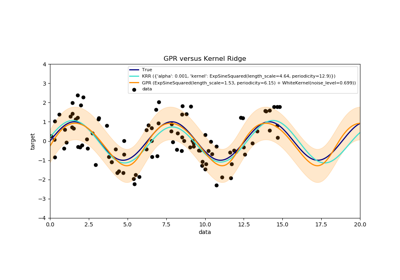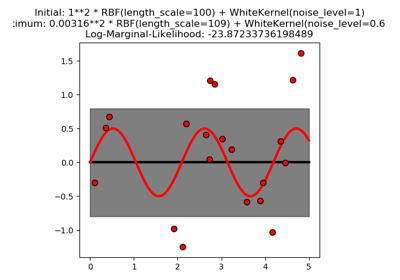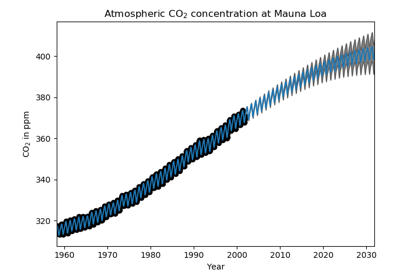# sklearn.gaussian_process.kernels.WhiteKernel¶

class sklearn.gaussian_process.kernels.WhiteKernel(noise_level=1.0, noise_level_bounds=(1e-05, 100000.0))[source]

White kernel.

The main use-case of this kernel is as part of a sum-kernel where it explains the noise-component of the signal. Tuning its parameter corresponds to estimating the noise-level.

k(x_1, x_2) = noise_level if x_1 == x_2 else 0

New in version 0.18.

Parameters: noise_level : float, default: 1.0 Parameter controlling the noise level noise_level_bounds : pair of floats >= 0, default: (1e-5, 1e5) The lower and upper bound on noise_level bounds Returns the log-transformed bounds on the theta. hyperparameter_noise_level hyperparameters Returns a list of all hyperparameter specifications. n_dims Returns the number of non-fixed hyperparameters of the kernel. theta Returns the (flattened, log-transformed) non-fixed hyperparameters.

Methods

 __call__(self, X[, Y, eval_gradient]) Return the kernel k(X, Y) and optionally its gradient. clone_with_theta(self, theta) Returns a clone of self with given hyperparameters theta. diag(self, X) Returns the diagonal of the kernel k(X, X). get_params(self[, deep]) Get parameters of this kernel. is_stationary(self) Returns whether the kernel is stationary. set_params(self, \*\*params) Set the parameters of this kernel.
__init__(self, noise_level=1.0, noise_level_bounds=(1e-05, 100000.0))[source]
__call__(self, X, Y=None, eval_gradient=False)[source]

Return the kernel k(X, Y) and optionally its gradient.

Parameters: X : array, shape (n_samples_X, n_features) Left argument of the returned kernel k(X, Y) Y : array, shape (n_samples_Y, n_features), (optional, default=None) Right argument of the returned kernel k(X, Y). If None, k(X, X) if evaluated instead. eval_gradient : bool (optional, default=False) Determines whether the gradient with respect to the kernel hyperparameter is determined. Only supported when Y is None. K : array, shape (n_samples_X, n_samples_Y) Kernel k(X, Y) K_gradient : array (opt.), shape (n_samples_X, n_samples_X, n_dims) The gradient of the kernel k(X, X) with respect to the hyperparameter of the kernel. Only returned when eval_gradient is True.
bounds

Returns the log-transformed bounds on the theta.

Returns: bounds : array, shape (n_dims, 2) The log-transformed bounds on the kernel’s hyperparameters theta
clone_with_theta(self, theta)[source]

Returns a clone of self with given hyperparameters theta.

Parameters: theta : array, shape (n_dims,) The hyperparameters
diag(self, X)[source]

Returns the diagonal of the kernel k(X, X).

The result of this method is identical to np.diag(self(X)); however, it can be evaluated more efficiently since only the diagonal is evaluated.

Parameters: X : array, shape (n_samples_X, n_features) Left argument of the returned kernel k(X, Y) K_diag : array, shape (n_samples_X,) Diagonal of kernel k(X, X)
get_params(self, deep=True)[source]

Get parameters of this kernel.

Parameters: deep : boolean, optional If True, will return the parameters for this estimator and contained subobjects that are estimators. params : mapping of string to any Parameter names mapped to their values.
hyperparameters

Returns a list of all hyperparameter specifications.

is_stationary(self)[source]

Returns whether the kernel is stationary.

n_dims

Returns the number of non-fixed hyperparameters of the kernel.

set_params(self, **params)[source]

Set the parameters of this kernel.

The method works on simple kernels as well as on nested kernels. The latter have parameters of the form <component>__<parameter> so that it’s possible to update each component of a nested object.

Returns: self
theta

Returns the (flattened, log-transformed) non-fixed hyperparameters.

Note that theta are typically the log-transformed values of the kernel’s hyperparameters as this representation of the search space is more amenable for hyperparameter search, as hyperparameters like length-scales naturally live on a log-scale.

Returns: theta : array, shape (n_dims,) The non-fixed, log-transformed hyperparameters of the kernel

## Examples using sklearn.gaussian_process.kernels.WhiteKernel¶Comparison of kernel ridge and Gaussian process regressionGaussian process regression (GPR) with noise-level estimationGaussian process regression (GPR) on Mauna Loa CO2 data.